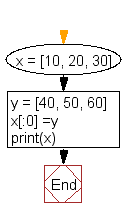﻿ Python: Extend a list without append - w3resource# Python: Extend a list without append

## Python List: Exercise - 68 with Solution

Write a Python program to extend a list without append.

Sample Solution:-

Python Code:

``````x = [10, 20, 30]
y = [40, 50, 60]
x[:0] =y
print(x)
```
```

Sample Output:

```[40, 50, 60, 10, 20, 30]
```

Pictorial Presentation:Flowchart:## Visualize Python code execution:

The following tool visualize what the computer is doing step-by-step as it executes the said program:

Python Code Editor:

Have another way to solve this solution? Contribute your code (and comments) through Disqus.

What is the difficulty level of this exercise?

Test your Python skills with w3resource's quiz

﻿

## Python: Tips of the Day

Floor Division:

When we speak of division we normally mean (/) float division operator, this will give a precise result in float format with decimals.

For a rounded integer result there is (//) floor division operator in Python. Floor division will only give integer results that are round numbers.

```print(1000 // 300)
print(1000 / 300)```

Output:

```3
3.3333333333333335```